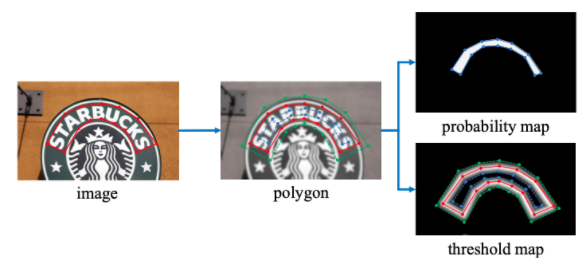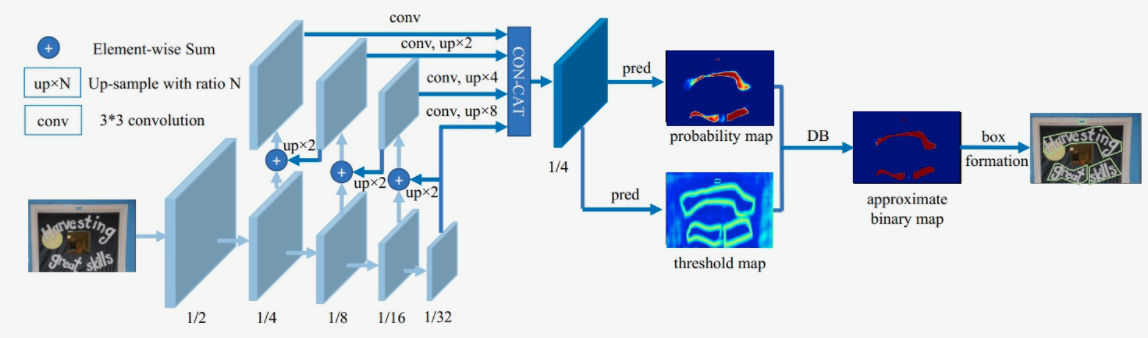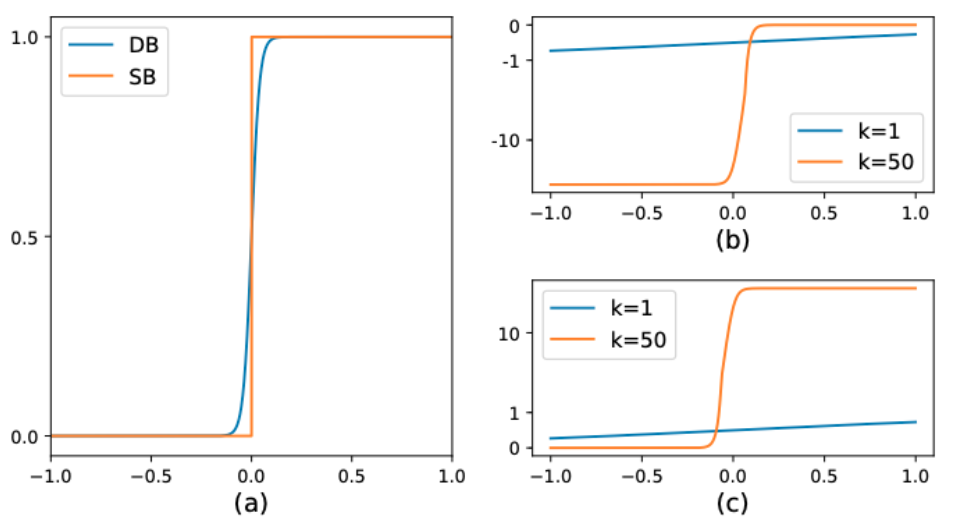# DBNet¶

## 背景介绍¶

DBNet对这个流程进行了优化，对每个像素点进行自适应二值化，二值化的阈值由网络学习得到，彻底将二值化这一步骤加入到网络里一起训练，这样最终的输出图对于阈值就会非常鲁棒。

## 模型输入标签¶

DB网络中，网络的输出为3个部分：概率图、阈值图和近似二值图：

• 概率图：图中每个像素点的值为该位置属于文本区域的概率。

• 阈值图：图中每个像素点的值为该位置的二值化阈值。

• 二值图：由概率图和阈值图通过DB算法计算得到，图中像素的值为0或1。## 模型介绍¶

DB文本检测模型使用了标准的FPN结构，网络结构如 图2 所示。1. 第一模块：使用CNN网络，对输入图像提取特征，得到特征图，本实验使用的骨干网络是轻量化网络MobileNetv3，同时使用了FPN结构，获取多尺度的特征，在本实验中，我们提取4个不同尺度下的特征图做拼接。

2. 第二模块：使用一个卷积层和两个转置卷积层的结构获取预测的概率图和阈值图；

3. 第三模块：使用DB方法获取近似二值图。

$\hat{B} = \frac{1}{1 + e^{-k(P_{i,j}-T_{i,j})}}$

$l_+ = -log(\frac{1}{1 + e^{-k(P_{i,j}-T_{i,j})}})$
$l_- = -log(1-\frac{1}{1 + e^{-k(P_{i,j}-T_{i,j})}})$

$\frac{\delta{l_+}}{\delta{x}} = -kf(x)e^{-kx}$
$\frac{\delta{l_-}}{\delta{x}} = -kf(x)$## 模型loss¶

$L = L_b + \alpha \times L_s + \beta \times L_t$

1）首先是Dice Loss，Dice Loss是比较预测结果跟标签之间的相似度，常用于二值图像分割。

$$dice\_loss = 1 - \frac{2 \times intersection\_area}{total\_area}$$

2）其次是MaskL1 Loss，是计算预测值和标签间的$$L_1$$距离

2）最后是Balance Loss，是带OHEM的Dice Loss，目的是为了改善正负样本不均衡的问题。OHEM为一种特殊的自动采样方式，可以自动的选择难样本进行loss的计算，从而提升模型的训练效果。

## 模型优缺点¶

### 优点¶

• 可以同时检测水平、多方向和弯曲文字；

• 在性能和速度上都获取不错的效果。